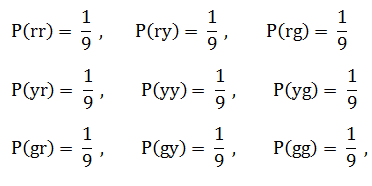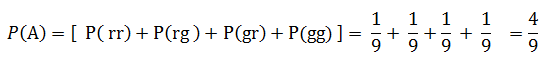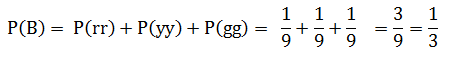# MA 121: Introduction to statistics coaching session 2.1.1 #11

11) Hi this is Asmita! I am a Student Success Coach and will be explaining some practice problems from the MA121 Introduction to Statistics course. Today we will review an assigned problem from Unit 2. In the “Homework Assessment” you were asked to solve this problem:

Assuming that each outcome is equally likely, find the probability of each event in Exercise 7.

We will use the concept of equally likely outcomes to find the probability of each outcomes.
Then we will approach this problem as follows:

1. Use the reference from exercise 7, and write down the given things.
2. Use the concept of probability of event to find the probability of event.
3. Find the required probability by plugging the individual probabilities.

By referring exercise 7, the sample space for the experiment of randomly drawing out, with replacement, two marbles in succession is
S = {rr, ry, rg, yr, yy, yg, gr, gy, gg}
where, ‘r’ denotes red marble, ‘y’ denotes yellow marble and ‘g’ denotes green marble.
“Equally likely outcomes” are the outcomes that have the same probability of occurring.
Sample space S has 9 outcomes. Since these nine outcomes are equally likely, which must add up to 1, each outcome has probability 1/9. That is,a.

Step 1: Write the given things from exercise 7:
Event A: No yellow marble is drawn.
Possible outcomes are A = {rr, rg, gr, gg}

Step 2: Find the probability of event A:
To find probability of event A, we find sum of probability of individual outcomes in event A. Therefore,
P(A) = [ P( rr) + P(rg ) + P(gr) + P(gg) ]

Step 3: Plug the individual probabilities:b.

Step 1: Write the given things from exercise 7:
Event B: The two marbles drawn have the same color.
Possible outcomes are B = {rr, yy, gg}

Step 2: Find the probability of event B:
To find the probability of event B, we find the sum of probability of individual outcomes in event B. Therefore,
P(B) = P(rr) + P(yy) + P(gg)

Step 3: Plug the individual probabilities:c.

Step 1: Write the given things from exercise 7:
An event C: At least one marble of each color is drawn.
There are no outcomes for this event; therefore, we write C= Ø.
This is called as empty set, and the event is called an impossible event.

Step 2: Find the probability of event C:
We have event C: Ø (empty set)
The probability of an empty set (impossible event) is 0.

Step 3:

P( C ) = 0

Conclusion:
a. The probability that no yellow marble is drawn is 4/9.
b. The probability that two marbles drawn have the same color is 1/3.
c. The probability that at least one marble of each color is drawn is 0.

In addition to this, I am also attaching the pdf document of the practice problem for your ready reference.
Question 11.pdf (388.8 KB)

Please let me know if you have any question on this problem or on this topic generally. I will be here in the forum for the next hour.